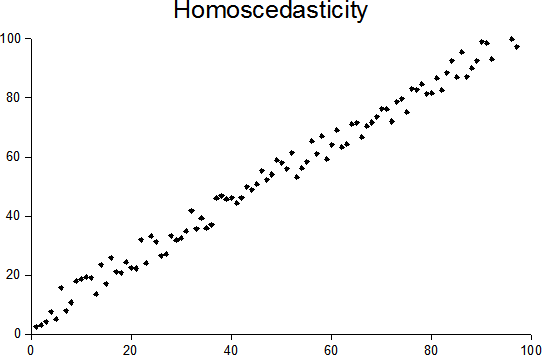# Goldfeld Quandt Test: Definition, Steps to Running

Statistics Definitions > Goldfeld Quandt Test

## What is the Goldfeld Quandt Test?Homoscedastic data is evenly dispersed around the line. Image: Sreebot| Wikimedia CommonsThe Goldfeld Quandt Test is a test used in regression analysis to test for homoscedasticity. It compares variances of two subgroups; one set of high values and one set of low values. If the variances differ, the test rejects the null hypothesis that the variances of the errors are not constant.

Although Goldfeld and Quandt described two types of test in their paper (parametric and non-parametric), the term “Quandt Goldfeld test” usually means the parametric test. The assumption for the test is that the data is normally distributed.

The test statistic for this test is the ratio of mean square residual errors for the regressions on the two subsets of data. This corresponds to the F-Test for equality of variances. Both the one-tailed and two-tailed tests can be used.

## Steps for Running the Test

1. Order the data in ascending order. This step depends upon you, the researcher, finding an appropriate way to order the sample. Usually, ordering by magnitude of x (one independent variable) works.
2. Divide your data into three parts*.
3. Drop the observations in the middle part.
4. Run separate regression analysis on the top and bottom parts (in other words, the groups with high values of x and low values of x). After each regression, find the Residual Sum of Squares.
5. Calculate the ratio of the Residual sum of squares, where RSS2 is the set of high values and RSS1 is the set of low values: (RSS2/df)/(RSS1/df).
6. Apply the conventional decision rule for an F-test. In general, large F values typically indicate that the variances are different.

*Goldfeld and Quandt did not specify how many central observations to remove. Using one third is optional, but recommended. If you increase the size of this middle portion, it will increase the power of the test. However, the degrees of freedom for the test statistic will be reduced. It’s also recommended that as the sample size increases, you decrease the size of this center part.

Reference:
S.M. Goldfeld & R.E. Quandt (1965), Some Tests for Homoskedasticity. Journal of the American Statistical Association 60, 539–547

CITE THIS AS:
Stephanie Glen. "Goldfeld Quandt Test: Definition, Steps to Running" From StatisticsHowTo.com: Elementary Statistics for the rest of us! https://www.statisticshowto.com/goldfeld-quandt-test/
---------------------------------------------------------------------------Need help with a homework or test question? With Chegg Study, you can get step-by-step solutions to your questions from an expert in the field. Your first 30 minutes with a Chegg tutor is free!